# 天池大数据竞赛——天池精准医疗大赛人工智能辅助糖尿病遗传风险预测赛后总结

## 天池大数据竞赛官方网址（链接）

#### 天池精准医疗大赛是我第一次正式参加与学习的数据竞赛，在这十几天的过程中，学习到很多参与这些数据竞赛的技巧和知识，虽然结果并不理想，但是总归是学习到不少。这篇文章也是梳理总结一下这段时间以来的经验和技术。在此需要感谢天池技术圈里的大佬共享的知识资源和Kaggle网站中共享的一些学习思路和资源。具体参考链接请见Reference。## 一、赛题背景介绍

### 2.赛题数据

#### 数据集中包含两个部分：训练集文件和测试集文件，部分字段名已经做脱敏处理，训练集中包含37个医学指标作为基本数据特征，每个文件的第一行是字段名，之后的每一行代表的是一个个体。文件共包含42个字段，包含数值型、字符型、日期型等众多数据类型，详细的字段名下数据类型请见表1，部分字段内容在部分人群中有缺失，其中第一列为个体ID号。训练文件的最后一列为标签列，即需要预测的目标血糖值。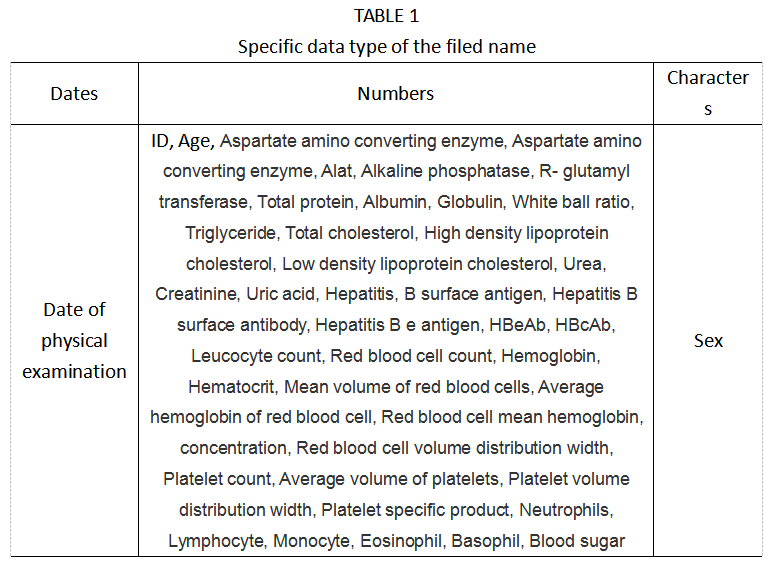#### 数据的大致格式如下图所示，选取其中十个人的数据的一部分作为展示。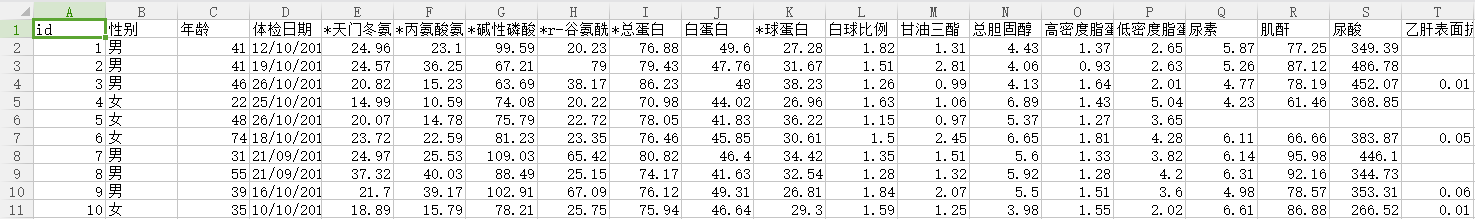## 二、数据统计信息

### 1.数据缺失比例统计

#### 数据的缺失比例，以数据的每个基本特征为单位，统计各列数据的缺失值的数目，并与该列数据总数据量相比，统计每个基本特征的缺失比例，便于下一步删除缺失比例较高的基本特征。数据集的各个基本特征缺失比例下图所示。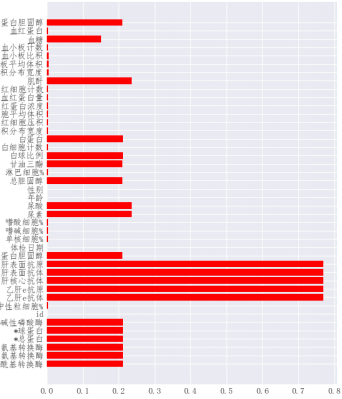### 1.数据特征影响权重

#### 数据特征影响权重，以LightGBM为核心算法，通过相关函数可以实现每个输入特征与预测结果之间的关联性，也就是数据特征对于预测结果的影响权重。通过这一比较结果，我们可以获知对于预测模型无效的数据特征，同时根据该结果，调整各个单模型的算法的基本参数，为模型调优提供参考依据。数据集的基本特征影响权重如下图所示。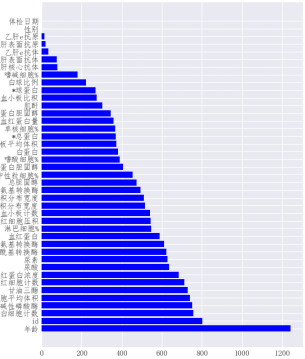### 3.基本特征与血糖值分布

#### 基本特征与血糖值的分布，在数据处理前，了解各个基本特征与血糖分布的整体情况，为特征工程提供直观的参考依据。对于训练集，将血糖值为Y轴坐标，各个基本特征为X轴坐标，做出各个基本特征与血糖值的分布情况，下图为R-谷氨酰基转换酶和血糖分布情况。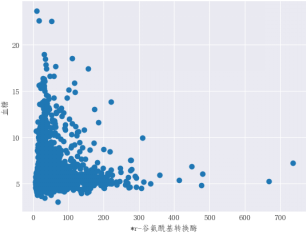### 4.特征先验高斯分布

#### 基本特征的先验高斯分布，是以先验的高斯分布去绘制血糖值与输入的基本特征之间的分布关系，在理想状态下，分布曲线应与相应的推理条件吻合，绘制基本特征的先验高斯分布，有助于后期数据的平滑处理。r-谷氨酰基转换酶和血糖的先验高斯分布图如图4所示，由图观察可以轻易得出，其概率分布与理想情况存在较大的偏离，因此需要对数据集的基本特征做平滑处理。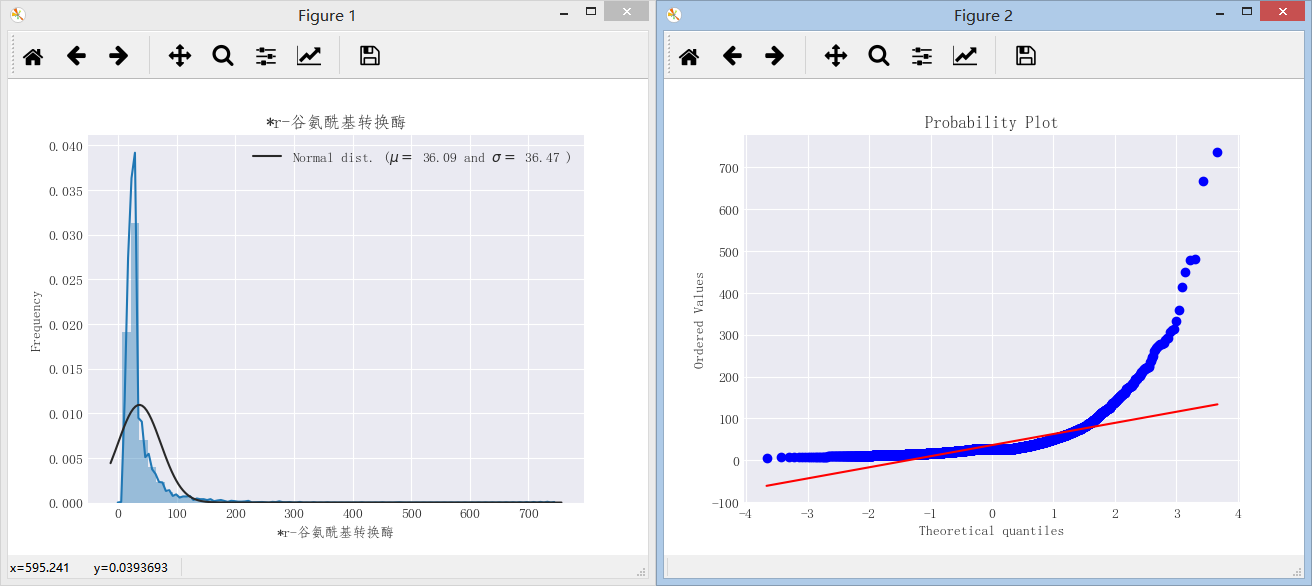### 5.特征关联热力图

#### 特征关联热力图采用的是Python中seaborn库进行操作，相关介绍请见seaborn绘制热力图坐标标签。## 三、数据预处理

### 2.去除离群值与无意义值

#### 去除对预测模型无影响的基本特征和离群值，是指删除在预测模型构建过程中，影响权重为零的基本特征，这些特征也符合常识的认知。由Section 2部分的工作可知，体检日期和性别这两个基本特征对于预测模型没有贡献，由基础医学认知也可认为体检日期和性别与血糖并无直接关系。离群值是指数据集中，明显与大部分的数据分布之间存在着极端的差距，离群值的存在往往会扭曲预测结果进而影响模型的精度。对于离群值可以用Box Plot来发现，一个典型的Box Plot是基于Upper Whisker, Upper Quartile, Median, Lower Quartile, Lower Whisker这五个值来计算得到。典型的Box Plot示意图如下图所示。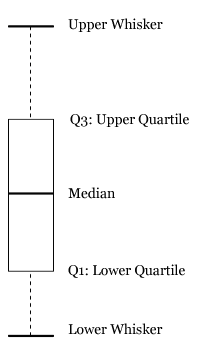### 4.特征平滑处理

#### 对数据集的基本特征做平滑处理，是由于数据集的基础特征的分布与推理条件中先验高斯分布之间存在较大的差异，如果数据集中的特征是不平滑的，这对于模型的拟合来说会有负面影响，同时有的噪声数据会影响拟合的函数的准确性，因此在特征工程工作和数据挖掘之前，先要对数据集进行平滑处理操作。值得一提的是，并非所有的基本特征都需要进行平滑处理，如淋巴细胞这一基本特征，其基本分布与推理条件中的先验高斯分布基本吻合，如下图所示。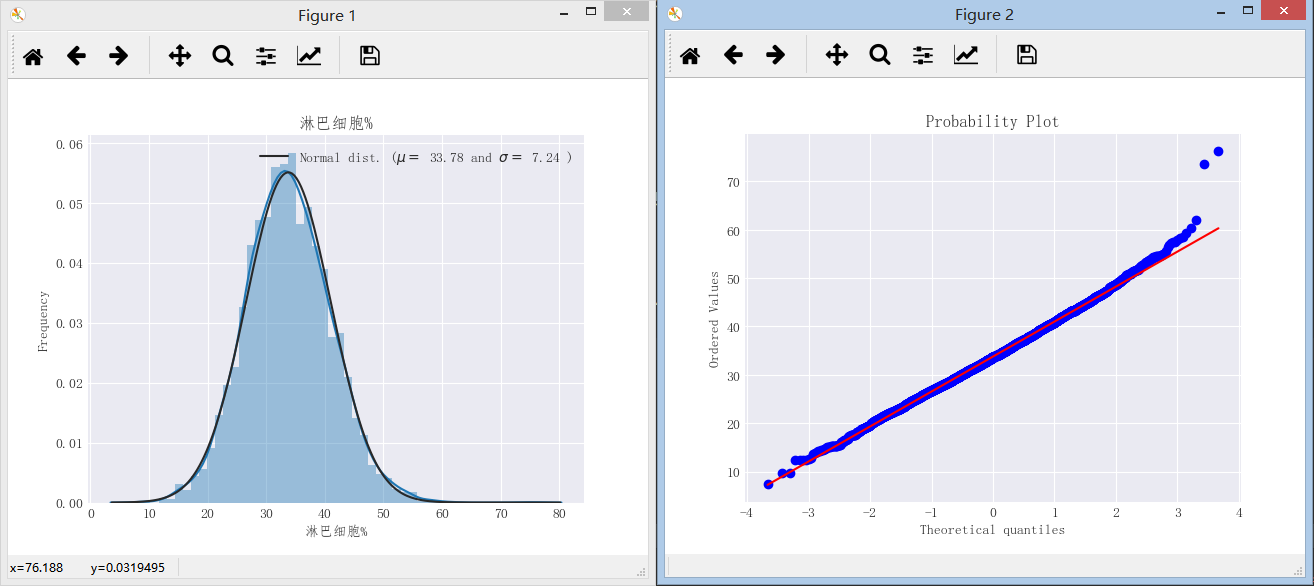## 四、数据特征工程

#### 本比赛中，由于源数据集的基本特征为医学特征，难以构建合理的新特征，本文选用多项式特征的方法完成特征工程部分工作。采用sklearn中专门产生多项式的函数PolynomialFeature产生相互影响的特征集。多项式特征的方法产生相互影响的数据集的过程可以用图8来作为演示，由本身的输入矩阵产生一个伪特征矩阵，由此可以用线性回归的方式来做非线性回归的预测，通过产生高维度的特征空间。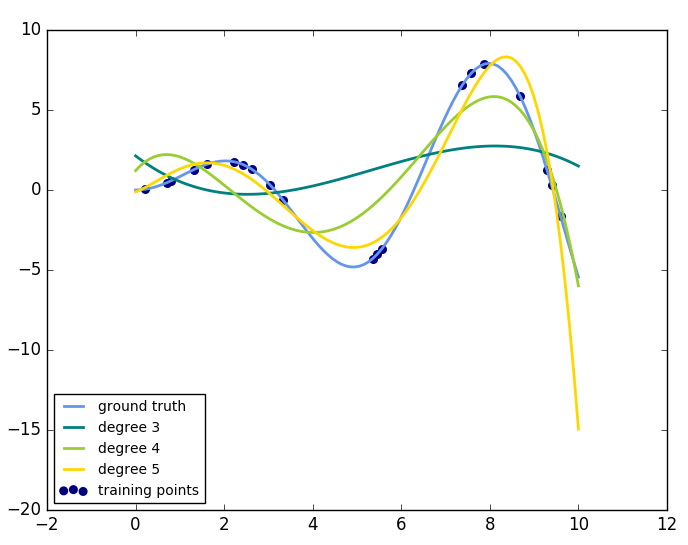## 五、代码部分

#### 赛题数据请见天池数据竞赛官网，根据实际情况适当调整代码。

# coding=utf-8
'''
Author:chenhao
Date: Jan 19 ,2017
Description: Data Visualization and Data Characteristics
'''
import time
import datetime
import numpy as np
import pandas as pd

import matplotlib.pyplot as plt
import seaborn as sns

import lightgbm as lgb
from dateutil.parser import parse
from sklearn.cross_validation import KFold
from sklearn.metrics import mean_squared_error

from pylab import mpl

from scipy import stats
from scipy.stats import norm, skew

import warnings
def ignore_warn(*args , **kwargs):
pass
warnings.warn = ignore_warn

pd.set_option('display.float_format',lambda x:'{:.3f}'.format(x))    #控制输出为精确到小数点后三位

color = sns.color_palette()
sns.set_style('darkgrid')

#导入数据
data_path = 'data/'
train = pd.read_csv(data_path + 'd_train_20180102.csv', encoding='gb2312')
test = pd.read_csv(data_path + 'd_test_A_20180102.csv', encoding='gb2312')

#将体检日期改写为天数的格式
train['体检日期'] = (pd.to_datetime(train['体检日期']) - parse('2017-09-10')).dt.days
test['体检日期'] = (pd.to_datetime(test['体检日期']) - parse('2017-09-10')).dt.days

#对性别进行转换，且其中男性比例为51%
train['性别'] = train['性别'].map({'男': 1, '女': 0, '??':0})
test['性别'] = test['性别'].map({'男': 1, '女': 0})
#print (train['性别'].describe())

#填充中位数
train_fill = train.drop(['id','性别','血糖'],axis=1)
train_fill.fillna(train_fill.median(axis=0), inplace=True)

#################################################################################################
#Step1：数据集各特征缺失比例及其图像
#################################################################################################

data = pd.concat([train,test],axis=0)
null_percentage = data.isnull().sum()/len(data)
print ('The null data percentage is:',null_percentage)

#显示各特征缺失比例图像

mpl.rcParams['font.sans-serif'] = ['FangSong']

null_percentage = null_percentage.reset_index()
null_percentage.columns = ['column_name','column_value']
ind = np.arange(null_percentage.shape)
fig , ax = plt.subplots(figsize = (6, 8))
rects = ax.barh(ind,null_percentage.column_value.values,color='red')
ax.set_yticks(ind)
ax.set_yticklabels(null_percentage.column_name.values,rotation='horizontal')
ax.set_xlabel("各基本特征缺失数据值")
plt.show()

#################################################################################################
#step2：各个特征对于血糖的影响程度(使用lightgbm对特征的影响因子进行排序)
#################################################################################################

def make_feat(train, test):
train_id = train.id.values.copy()
test_id = test.id.values.copy()
#对数据进行合并与重塑
data = pd.concat([train, test])

data['性别'] = data['性别'].map({'男': 1, '女': 0})
data['体检日期'] = (pd.to_datetime(data['体检日期']) - parse('2017-9-10')).dt.days

data.fillna(data.median(axis=0), inplace=True)

train_feat = data[data.id.isin(train_id)]
test_feat = data[data.id.isin(test_id)]

return train_feat, test_feat

importance = train.drop(['血糖'],axis=1)
importance_name = importance.columns

train_feat, test_feat = make_feat(train, test)

predictors = [f for f in test_feat.columns if f not in ['血糖']]

def evalerror(pred, df):
label = df.get_label().values.copy()
score = mean_squared_error(label, pred) * 0.5
return ('0.5mse', score, False)

print('开始训练...')
params = {
'learning_rate': 0.01,
'boosting_type': 'gbdt',
'objective': 'regression',
'metric': 'mse',
'sub_feature': 0.7,
'num_leaves': 60,
'colsample_bytree': 0.7,
'feature_fraction': 0.7,
'min_data': 100,
'min_hessian': 1,
'verbose': -1,
}

print('开始CV 5折训练...')
t0 = time.time()
train_preds = np.zeros(train_feat.shape)
#产生相应的id数为行数5列全零数据
test_preds = np.zeros((test_feat.shape, 5))
kf = KFold(len(train_feat), n_folds=5, shuffle=True, random_state=520)
for i, (train_index, test_index) in enumerate(kf):
print('第{}次训练...'.format(i))
train_feat1 = train_feat.iloc[train_index]
train_feat2 = train_feat.iloc[test_index]
lgb_train1 = lgb.Dataset(train_feat1[predictors], train_feat1['血糖'], categorical_feature=['性别'])
lgb_train2 = lgb.Dataset(train_feat2[predictors], train_feat2['血糖'])
gbm = lgb.train(params,
lgb_train1,
num_boost_round=3000,
valid_sets=lgb_train2,
verbose_eval=100,
feval=evalerror,
early_stopping_rounds=100)
feat_imp = pd.Series(gbm.feature_importance(), index=predictors).sort_values(ascending=False)
train_preds[test_index] += gbm.predict(train_feat2[predictors])
test_preds[:, i] = gbm.predict(test_feat[predictors])
print('线下得分：    {}'.format(mean_squared_error(train_feat['血糖'], train_preds) * 0.5))
print('CV训练用时{}秒'.format(time.time() - t0))

print(feat_imp)

mpl.rcParams['font.sans-serif'] = ['FangSong']

feat_imp = feat_imp.reset_index()
feat_imp.columns = ['column_name','column_value']
ind = np.arange(feat_imp.shape)
fig , ax = plt.subplots(figsize = (6,8))
rects = ax.barh(ind,feat_imp.column_value.values,color='blue')
ax.set_yticks(ind)
ax.set_yticklabels(feat_imp.column_name.values,rotation='horizontal')
ax.set_xlabel("各个基本特征影响权重")

plt.show()

#################################################################################################
#step3：各个特征对于血糖的直接影响关系(选取其中一个特征作为代表)
#################################################################################################

mpl.rcParams['font.sans-serif'] = ['FangSong']
mpl.rcParams['axes.unicode_minus'] = False
fig , ax = plt.subplots()
ax.scatter(x=train_fill['*天门冬氨酸氨基转换酶'],y=train['血糖'])
plt.ylabel('血糖')
plt.xlabel('*天门冬氨酸氨基转换酶')

soft , b = stats.boxcox(train_fill['*天门冬氨酸氨基转换酶'])
#soft += 2
fig , ax = plt.subplots()
ax.scatter(x= soft,y=train['血糖'])
plt.ylabel('血糖')
plt.xlabel('*天门冬氨酸氨基转换酶')

print(soft)
print(b)
plt.show(1)
plt.show(2)

#################################################################################################
#step4：各个特征对于血糖的先验高斯分布绘制图像比较
#将所有特征的基本分布情况进行展示
#################################################################################################
mpl.rcParams['font.sans-serif'] = ['FangSong']
mpl.rcParams['axes.unicode_minus'] = False

#sns.distplot(train_fill['白蛋白'],fit=norm)
#(mu,sigma) = norm.fit(train_fill['白蛋白'])
#print('\n mu = {:.2f} and sigma = {:.2f}\n'.format(mu, sigma))
#plt.legend(['Normal dist. ($\mu=$ {:.2f} and $\sigma=$ {:.2f} )'.format(mu, sigma)], loc='best')
#plt.ylabel('Frequency')
#plt.title('血糖分布')
#fig1 = plt.figure()
#res1 = stats.probplot(train_fill['白蛋白'], plot=plt)

#soft , b = stats.boxcox(train_fill['*天门冬氨酸氨基转换酶'])

#train_fill['性别_log'] = np.log(train_fill['性别'])
#train_fill['*r-谷氨酰基转换酶_log'] , a = stats.boxcox(train_fill['*r-谷氨酰基转换酶'])

dist = sns.distplot(train_fill['嗜碱细胞%'],fit=norm)
(mu,sigma) = norm.fit(train_fill['嗜碱细胞%'])
print('\n mu = {:.2f} and sigma = {:.2f}\n'.format(mu, sigma))
plt.legend(['Normal dist. ($\mu=$ {:.2f} and $\sigma=$ {:.2f} )'.format(mu, sigma)], loc='best')
plt.ylabel('Frequency')
plt.title('嗜碱细胞%')
fig = plt.figure()
res = stats.probplot(train_fill['嗜碱细胞%'], plot=plt)

#a = train.loc[train[train['性别'] == 0 ].index]
#print(a)

plt.show()

#################################################################################################
#step5：尝试调用scipy中的coxbox函数对数据进行平滑处理
#################################################################################################

soft , b = stats.boxcox(train_fill['甘油三酯'])

mpl.rcParams['font.sans-serif'] = ['FangSong']
mpl.rcParams['axes.unicode_minus'] = False

sns.distplot(soft,fit=norm)
(mu,sigma) = norm.fit(soft)
print('\n mu = {:.2f} and sigma = {:.2f}\n'.format(mu, sigma))
plt.legend(['Normal dist. ($\mu=$ {:.2f} and $\sigma=$ {:.2f} )'.format(mu, sigma)], loc='best')
plt.ylabel('Frequency')
plt.title('血糖分布')
fig = plt.figure()
res = stats.probplot(soft, plot=plt)

plt.show()

#################################################################################################
#step6: 探索数据关联性特征
#################################################################################################
mpl.rcParams['font.sans-serif'] = ['FangSong']
mpl.rcParams['axes.unicode_minus'] = False

corrmat = train.corr()
f,ax = plt.subplots(figsize=(15,12))
ax.set_xticklabels(corrmat,rotation='horizontal')
sns.heatmap(corrmat, vmax =0.9,square=True)
label_y = ax.get_yticklabels()
plt.setp(label_y , rotation = 360)
label_x = ax.get_xticklabels()
plt.setp(label_x , rotation = 90)

plt.show()

#################################################################################################
#step7: 相关变量之间的散点图
#################################################################################################
mpl.rcParams['font.sans-serif'] = ['FangSong']
mpl.rcParams['axes.unicode_minus'] = False

sns.set()
cols = ['年龄','白细胞计数','甘油三酯','红细胞平均血红蛋白浓度','尿素','尿酸']
sns.pairplot(train_fill[cols],size=2.5)
plt.show()

#################################################################################################
#step7: 各变量的离群值查看
#################################################################################################
#train = train.drop(train[train['*r-谷氨酰基转换酶'] > 550].index)
#train = train.drop(train[train['*丙氨酸氨基转换酶'] == 388.0].index)
a = train.loc[train[train['红细胞平均血红蛋白浓度'] > 425].index]
a = a['血糖']
print(a)

#max = a.max()
#print(max)

#################################################################################################
#step8：各个特征对于血糖的直接影响关系(选取其中一个特征作为代表)
#################################################################################################

mpl.rcParams['font.sans-serif'] = ['FangSong']
mpl.rcParams['axes.unicode_minus'] = False
fig , ax = plt.subplots()
ax.scatter(x=train_fill['*天门冬氨酸氨基转换酶'],y=train['血糖'])
plt.ylabel('血糖')
plt.xlabel('*天门冬氨酸氨基转换酶')

soft , b = stats.boxcox(train_fill['*天门冬氨酸氨基转换酶'])
#soft += 2
fig , ax = plt.subplots()
ax.scatter(x= soft,y=train['血糖'])
plt.ylabel('血糖')
plt.xlabel('*天门冬氨酸氨基转换酶')

print(soft)
print(b)
plt.show(1)
plt.show(2)

## Reference

####  寒武纪之糖尿病血糖值预测的数据初探（肆），网址链接。